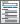»  Home »  Products »  Features »  Structural equation modeling (SEM)

## Structural equation modeling (SEM)

Estimate mediation effects, analyze the relationship between an unobserved latent concept such as depression and the observed variables that measure depression, model a system with many endogenous variables and correlated errors, or fit a model with complex relationships among both latent and observed variables. Fit models with continuous, binary, count, ordinal, fractional, and survival outcomes. Even fit multilevel models with groups of correlated observations such as children within the same schools. Evaluate model fit. Compute indirect and total effects. Fit models by drawing a path diagram or using the straightforward command syntax.

Learn about structural equation modeling (SEM).

Model specification

• Drag, drop, and connect to create path diagrams
• Estimate models from path diagrams
• Display results on the path diagram
• Save and modify diagrams
• Tools to create measurement and regression components
• Set constant and equality constraints by clicking
• Complete control of how your diagrams look

Additional classes of models for generalized SEMLinear and generalized-linear responsesMultilevel models• Two-, three-, and higher-level structural equation models
• Multilevel mixed-effects models
• Random intercepts and random slopes
• Crossed and nested random effects

Estimation methods for linear SEM• ML—maximum likelihood
• MLMV—maximum likelihood for missing values; sometimes called FIML
• ADF—asymptotic distribution free, meaning GMM (generalized method of moments) using ADF weighting matrix

Estimation methods for generalized SEM• Maximum likelihood
• Laplace approximation
• OIM—observed information matrix
• EIM—expected information matrix
• Satorra—Bentler estimator
• Robust—distribution-free linearized estimator
• Cluster–robust—robust adjusting for correlation within groups of observations
• Bootstrap—nonparametric bootstrap and clustered bootstrap
• Jackknife—delete-one, delete-n, and clustered jackknife
• Sampling weights and stage-level weights
• Stratification and poststratification
• Clustered sampling at one or more levels
• View and run all postestimation features for your command
• Automatically updated as estimation commands are run

Summary statistics data (SSD)Starting values• Automatic normalization (anchoring) constraints provide scale for latent variables; may be overridden
• May specify fraction of variance not due to measurement errorDirect and indirect effects for linear SEM• Confidence intervals
• Unstandardized or standardized units

Overall goodness-of-fit statistics for linear SEM• Model vs. saturated
• Baseline vs. saturated
• RMSEA, root mean squared error of approximation
• AIC, Akaike's information criterion
• BIC, Bayesian information criterion
• CFI, comparative fit index
• TLI, Tucker–Lewis index, a.k.a. nonnormed fit index
• SRMR, standardized root mean squared residual
• CD, coefficient of determination

Equation-level goodness-of-fit statistics for linear SEM• R-squared
• Equation-level variance decomposition
• Bentler–Raykov squared multiple-correlation coefficient

Group-level goodness-of-fit statistics for linear SEM• SRMR
• CD
• Model vs. saturated chi-squared contribution

Residual analysis for linear SEM• Mean residuals
• Variance and covariance residuals
• Raw, normalized, and standardized values available

Parameter tests

Group-level parameter tests• Group invariance by parameter class or user specified

Linear and nonlinear combinations of estimated parametersAssess nonrecursive system stability• Observed endogenous variables
• Latent endogenous variables
• Latent variables (factor scores)
• Equation-level first derivatives
• In- and out-of-sample prediction; may estimate on one sample and form predictions in another

Predictions for generalized SEM• Means of observed endogenous variables—probabilities for 0/1 outcomes, mean counts, etc.
• Linear predictions of observed endogenous variables
• Latent variables using empirical Bayes means and modes
• Standard errors of empirical Bayes means and modes
• Observed endogenous variables with and without predictions of latent variables
• Density function
• Distribution function
• Survivor function
• Predict observed endogenous variables marginally with respect to latent variables
• User-defined nonlinear predictions

Results

Factor variables with generalized SEM• Automatically create indicators based on categorical variables
• Form interactions among discrete and continuous variables
• Include polynomial terms
• Perform contrasts of categories/levels

Marginal analysisContrasts for generalized SEM• Analysis of main effects, simple effects, interaction effects, partial interaction effects, and nested effects
• Comparisons against reference groups, of adjacent levels, or against the grand mean
• Orthogonal polynomials
• Helmert contrasts
• Custom contrasts
• ANOVA-style tests
• Contrasts of nonlinear responses
• Balanced and unbalanced data
• Contrasts of means, intercepts, and slopes
• Graphs of contrasts
• Interaction plots

Pairwise comparisons for generalized SEM• Compare estimated means, intercepts, and slopes
• Compare marginal means, intercepts, and slopes
• Balanced and unbalanced data
• Nonlinear responses
• Multiple-comparison adjustments: Bonferroni, Sidak, Scheffe, Tukey HSD, Duncan, and Student–Newman–Keuls adjustments
• Group comparisons that are significant
• Graphs of pairwise comparisons

Explore more about SEM in Stata.